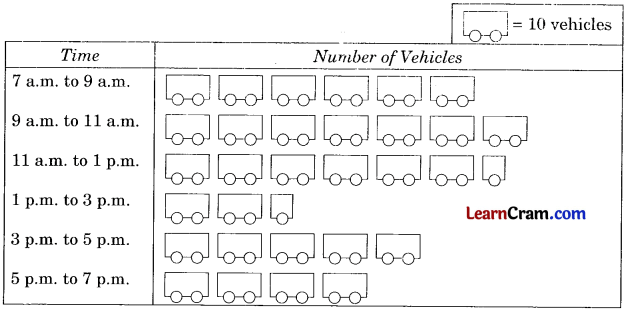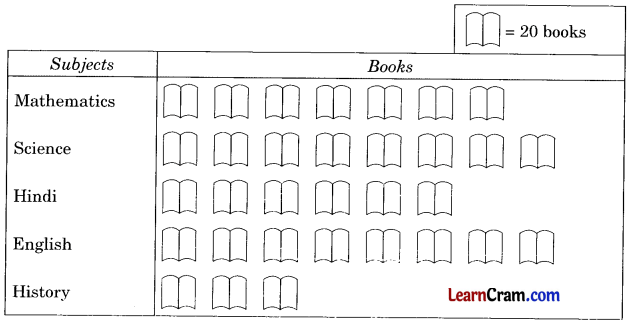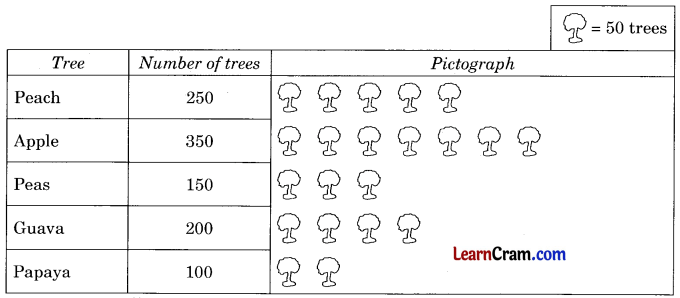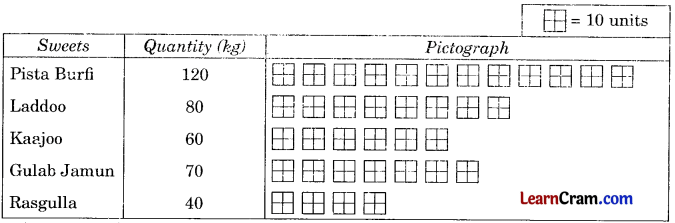# DAV Class 6 Maths Chapter 16 Worksheet 2 Solutions

The DAV Class 6 Maths Book Solutions and DAV Class 6 Maths Chapter 16 Worksheet 2 Solutions of Statistics offer comprehensive answers to textbook questions.

## DAV Class 6 Maths Ch 16 WS 2 Solutions

Question 1.
Given below is the pictograph showing the number of vehicles passing through a busy crossing in Delhi at different times of a particular day.(a) How many vehicles passed the crossing from 7 a.m. to 9 a.m.?
From 7 a.m. to 9 a.m., the number of vehicles passed the crossing is 60.

(b) How many vehicles passed the crossing from 11 a.m to 1 p.m.?
Number of Vehicles passed the crossing from 11 a.m. to 1 p.m. is 65.

(c) During which time of the day was traffic maximum?
Maximum traffic passed through the crossing is from 9 a.m. to 11. a.m.

(d) How many vehicles passed the crossing from 3 p.m. to 7 p.m.?
Number of vehicles passed through the crossing from 3 p.m. to 7 p.m. is 90.Question 2.
The following pictograph shows the number of books in different subjects in a library.(a) How many science books are there in the library?
8 × 20 = 160 books.

(b) Which subject books are maximum in the library?
Science and English books are maximum in the library.

(c) What is the total number of books in the library?
32 × 20 = 640 books.

(d) What is the total number of English and Hindi books?
14 × 20 = 280 books.

(e) How many more English books are there than Mathematics books?
160 – 140 = 20 books.

Question 3.
The number of different trees in an orchard are given below. Draw a pictograph to represent the given data. The first column is done for you.

 Tree Number of trees Peach 250 Apple 350 Peas 150 Guava 200 Papaya 100Question 4.
Quantities of different varieties of sweets sold on a festival day in a shop is given below. Prepare a pictograph. Also form any 2 questions on the pictograph.

 Sweets Quantity (K.g.) Pista Burfi 120 Laddoo 80 Kaajoo 60 Gulab Jamun 70 Rasgulla 40